## What is dags

dags provides tools to combine several interrelated functions into one function. The order in which the functions are called is determined by a topological sort on a Directed Acyclic Graph (DAG) that is constructed from the function signatures.## First example

Before talking about applications of dags, I will quickly show how it works on a toy example. Take for example, the following functions.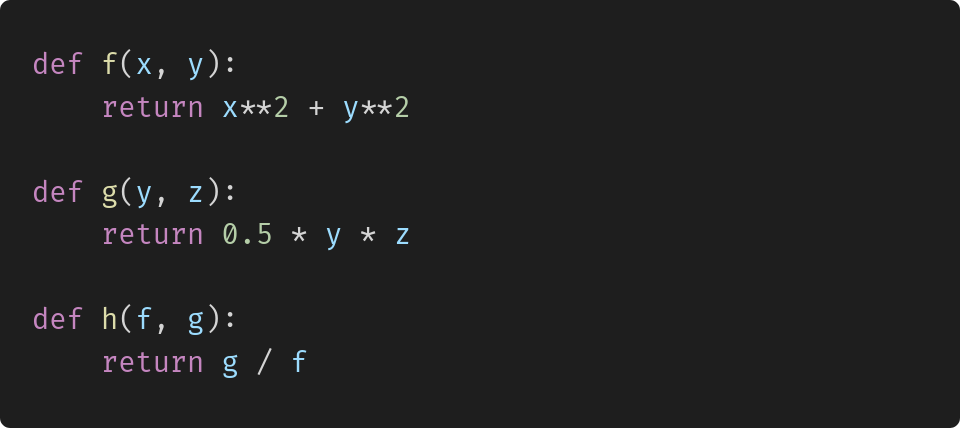Now assume that we are actually interested in a combined function that calculates h given x, y, and z. We could write this function manually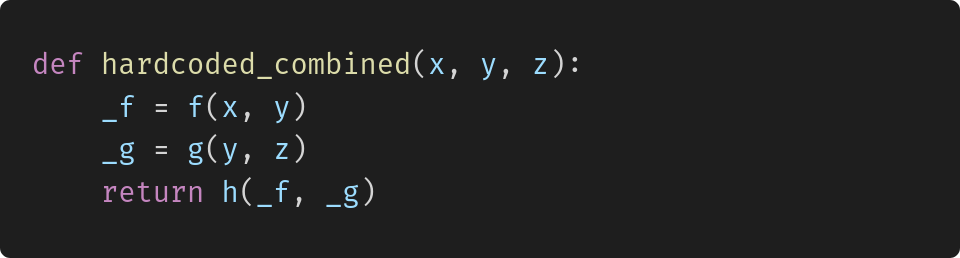But we can also create the function dynamically with dags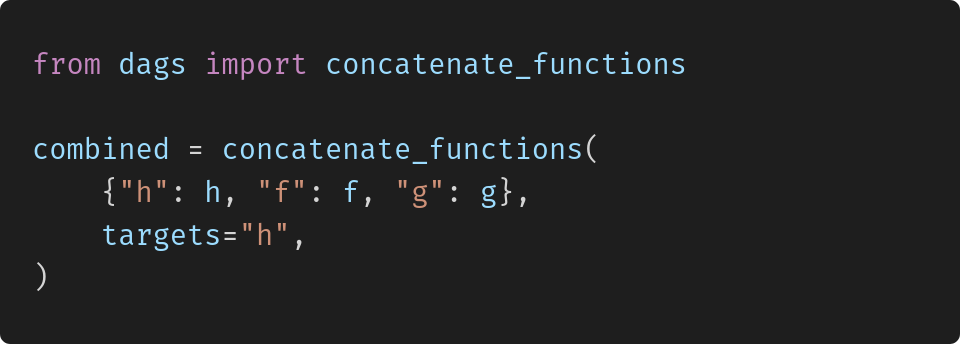hardcoded_combined and combined behave exactly the same and both return 0.6 when called with arguments x=1, y=2, and z=3.

You can find more examples in the documentation.

## How is dags useful?

On a first glance, this looks just like a complicated way of achieving something simple. So when could this be useful? Let’s look at three different situations in which dags can be really helpful.

#### 1. Complicated relationships between functions

In the above example, it was easy to figure out that it does not matter if f or g is called first, but h can only be called in the end. But what if you have dozens or hundreds of functions and the dependencies are more complicated?

A primary example of such a convoluted set of functions is the German tax and transfer system. If you don’t believe me, convince yourself.

The GErman Tax and Transfer SIMulator (GETTSIM) is an effort by several German universities and the major economics research institutes to replace custom codes at each institute by a clean and well tested Python implementation.

To achieve this, GETTSIM implements Python functions for all kinds of taxes and transfers and uses dags to figure out in which order these functions should be called.

In fact, dags was created by Tobias Raabe and me, while we worked on the architecture of GETTSIM.

#### 2. Need to exchange parts of the system

Another motivation for using dags over a hard-coded approach arises when it is necessary to easily switch out certain components of the system. To see how this situation arises in practice, let’s assume an economist wants to calculate the aggregate cost of replacing the current income tax by a simpler policy where everyone pays 25 % of their income. Keeping all other policies unchanged.

Assume the old and new income tax functions look as follows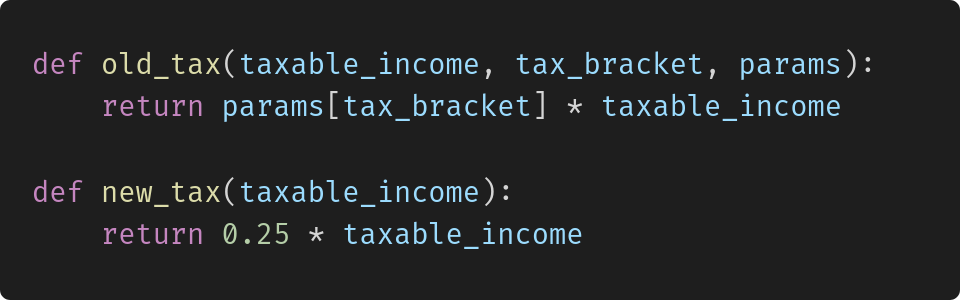Coding up the new function was simple enough, but to answer the research question we need to take into account all ramifications (e.g. changes in eligibility for different transfers due to changes in net income).

If the function that calculates aggregate taxes and transfers was hard-coded, we would roughly have to do the following:

• Add a flag that decides whether the new or old policy is active
• Pass the flag through to the point where the income tax is calculated
• Use the flag to switch between calling the old and new function

This is clearly not a desirable thing to do, especially if multiple researchers use the same code base to work on different questions.

Dags provides a much better way. Assume that old_functions is a dictionary containing all functions of the current tax and transfer system. Then, implementing the policy becomes as simple as: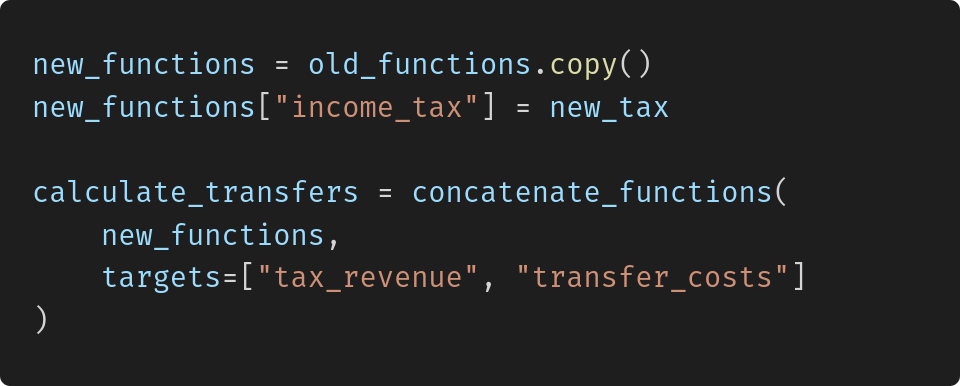It is not even a problem that the new income tax has a different signature than the old one. It could depend on more or fewer arguments as long as all of them are calculated by some function in the functions dictionary.

#### 3. Different combinations of functions are needed.

With dags it is easy to generate different combinations of a set of functions. Let’s first go back to the simple example to see how it is done: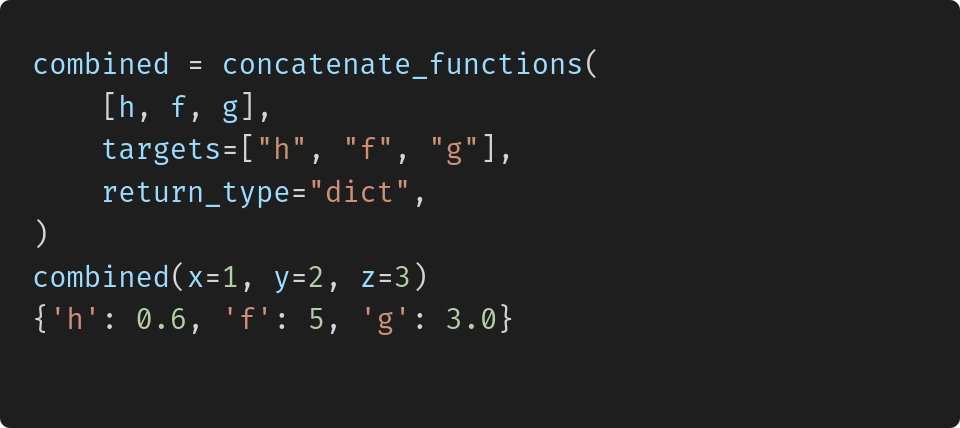By passing in a list of targets instead of just "h", we told dags that the combined function also needs to return the intermediate variables. By specifying return_type dict, we said that we want them in a dictionary. Other possible return types are "tuple" or "list".

Again, a practical application can be found in GETTSIM, where we sometimes need all taxes, transfers, and intermediate variables and sometimes we focus on a single variable (e.g. child benefits).

Not only can dags create a function that returns exactly what a user needs but it will also skip unnecessary computations. Only functions that are needed to create the targets will be called.

## Efficiency of dags vs hard-coding

Everything that is related to creating the dag and figuring out the order of execution is only done once, when the combined function is created and has no additional cost at runtime. Thus it can be seen as a compile time that is negligible if the combined function is called repeatedly.

The runtime of the dynamically created function is approximately the same as in a hard-coding approach.

## JAX compatibility

A very important feature of dags is that as long as the component functions are all jax compatible, the resulting function is also jax compatible. More precisely, dags created functions can be:

• Just in time compiled using jax.jit
• Vectorized, using jax.vmap
• Differentiated, using jax.grad and others.

In extensive benchmarks, we could not find any speed difference between a hard-coded and dags approach when using jax.jit or jax.vmap!